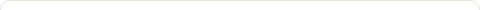# Java Program to find prime number upto N number

Posted on: March 13, 2016

Objectives :

• Write a Java program to find prime number upto N number
• Write a Java program to print prime number upto N number
• Write a Java program to find prime number upto N number using Command Line Arguments
• Write a Java Program to find prime number upto N number via Command Line Arguments
• Write a Java program to find prime number upto N number using Scanner class

Java Program / Code :

Method 1 : Java Program to find Prime Number upto N number using Scanner class

```
import java.util.Scanner;
{
public static void main(String[] args)
{
int n,p;
Scanner s=new Scanner(System.in);
System.out.println(“Enter number : ”);
n=s.nextInt();
for(int i=2;i<n;i++)
{
p=0;
for(int j=2;j<i;j++)
{
if(i%j==0)
p=1;
}
if(p==0){
System.out.println(i);
}
}
}
}
```

Method 2 : Java Program to find Prime Number upto N number using Scanner class and writing function to find prime number

Method isPrime() for checking if a number is prime or not

```public class PrimeNumber{
public boolean isPrime(int num) {
if ( num < 2 ){
return false;
}
for (int i = 2; i <= Math.sqrt(num); i++) {
if ( num % i == 0 ) {
return false;
}
}
return true;
}
}

// Mock Test Class to test above code
import java.util.Scanner;

public class Demo
{
public static void main(String[] args)
{
Scanner scanner = new Scanner(System.in);

int num = scanner.nextInt();

if ( num < 2 ) {
System.out.println("\n There are no Prime Numbers available");
System.exit(0);
}
System.out.println("\n Prime Numbers from 1 to "+ num);

for (int i = 3; i <= num; i++) {
System.out.print(", " + i);
}
}
}
}
```

Method 3 : Java Program to find Prime Number upto N number using Command line input

```
{
public static void main(String[] args)
{
int n,p;
n=Integer.parseint(args);
for(int i=2;i&lt;n;i++)
{
p=0;
for(int j=2;j&lt;i;j++)
{
if(i%j==0)
p=1;
}
if(p==0){
System.out.println(i);
}
}
}
}
```

Steps to run above program via command line :

2. Interpretation : C:\JavaPrograms>java PrimeNumber 20

Output :

2

3

5

7

11

13

17

19

### 1 Response to "Java Program to find prime number upto N number"for(int i=2 ; i & lt ; n ; i++)
{
p=0;
for(int j=2;j<i;j++)
what is this lt here?

Like